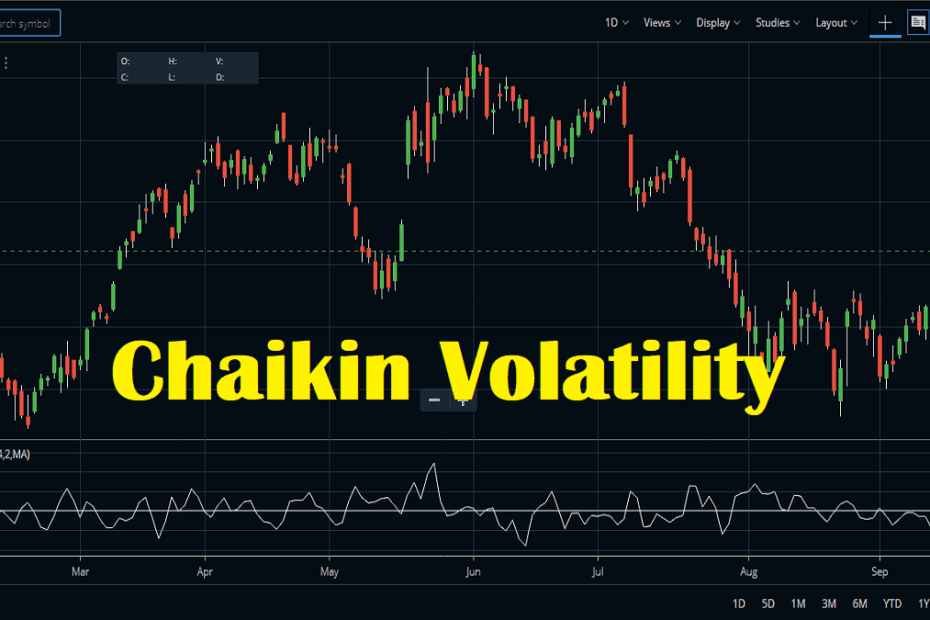Home » Blog » Trading » Technical Indicators » Chaikin Volatility Indicator Formula, Strategy

# Chaikin Volatility Indicator Formula, StrategyChaikin Volatility Indicator or CVI was developed by Marc Chaikin. He also has developed the Chaikin Money Flow or CMF indicator. Volatility is depicted by the indicator because it calculates the difference between the high and low for every period or candle.

## Some Common FAQ

How do you use Chaikin Volatility Indicator?

This indicator measures the difference between two moving averages of volume-weighted accumulation/ distribution line. Thus it differs from the Average True Range or ATR because it does not take into account the price gaps. When the indicator picks, the price retreats from a new high. A sharp rise in volatility indicators occurs before the market makes a new high. Sharp fall in volatility indicates that a reversal is likely.

What are the volatility indicators?

The volatility indicators indicate how volatile a stock, index or commodity is at a given point of time. The more volatile a stock, the more frequent ups and dows happen in its price. When the volatility is high, the stock’s daily range is also high. On the other hand, when the volatility is low, the stock’s daily range is also low.

## Chaikin Volatility Indicator Formula

This indicator is a volatility indicator that calculates the exponential moving average of the difference between the current period’s high and low prices and its value in a number of periods previously. A sudden increase indicates a directional move.

## How to set up Chaikin Volatility indicator on the charts?

### Chaikin Volatility Indicator in Zerodha Kite

• Open the MarketWatch on Zerodha Kite.
• Select your stock and right-click on it and select the chart icon.
• Open the chart window of the stock.
• Go to Chaikin Volatility from the Studies and click on it.
• The default parameters window will open where you can select the parameters.
• By default, the period is 14 and the rate of change is 2 and the moving average type is simple.

### Chaikin Volatility Indicator in Upstox Pro

Just like Zerodha, this indicator is available in the Upstox Pro trading terminal. Here also the default parameter is 14 period. The rate of change is 2 and the moving average type is simple.

## Chaikin Volatility Indicator Trading Strategy

CVI provides us with a measurement of volatility based on the range between the high price and the low price. A much broader range means the volatility is high. This indicator helps to identify potential tops and bottoms. Like the ATR it does not takes into account the gaps in the chart. This indicator is an oscillator and it oscillates above and below the zero line. Simply speaking above 0 is considered as more volatile and below 0 is considered as less volatile.

### #1 The basic trading strategies

In general, the basic signals are the increased volatility in a bull market denotes a potential market top. And a decreased volatility with a fall in price denotes a potential bottom.

As per the advance signals, in case the increase of volatility within a bear market is in a short time, the bottom can be very near. And if the decrease of volatility is over a longer period in a bull market, it denotes a potential end of the bull market.